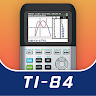# Real 84 ti Graphing Calculator - 83 ti Plus

## Information

 Updated 2019-10-24 Rating 4.6 ★ / 5 version 4.3.4-24-10-2019-12-release Size 15.81 MB OS Require Android 4.1.x - Jelly Bean Packagename com.calc.graph.ti84.ti83 Author's Notes Simulate for ti 83, ti 83 plus, ti 84, ti 84 plus, or calculator 570, 991

## Description

This calculator is designed for users use ti 84 Calculator, math helper.

This is an emulator of some models:
+ Free graphing calculator ti 83, ti 84 plus free, math algebra & calculus.
+ Free graphing calculator ti84 plus
+ Graphing calculator grafncalc83
+ Calculator advanced 570 fx es plus
+ calculator engineering 991 fx es plus
+ Calculator 991 ex plus t1-84
+ Calculator graphncalc 570 ex plus
+ Almost features of calculatorfree ti 89 and wabbit calculator
+ Graphic calculator can draw function, polar, parametric graph

+ Math formulas and physics formulas
+ Unit converter
+ Programming
+ Equation solver
+ Fraction calculation
+ Solve math problem by math camera, math solver
+ Statistic, linear algebra and symbolic calculation

Real 84 ti Graphing Calculator - 83 ti Plus APK - v4.3.4-24-10-2019-12-release

Updated: 2019-10-25

## What's new

Quick tile on status bar
Graph simultaneous plotting
Graph independent zoom
Statistical functions and documentation
Distribution calculation
randNorm, randBin and round functions
Bug fixes and stability improvement

## Rating

4.6
86 Total
5★ 69
4★ 10
3★ 1
2★ 0
1 ★ 6